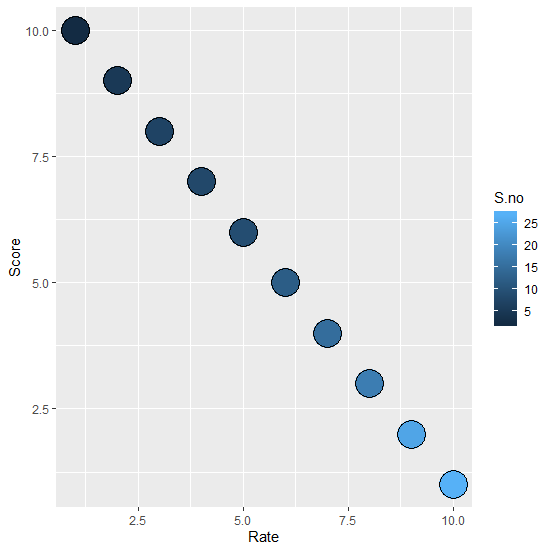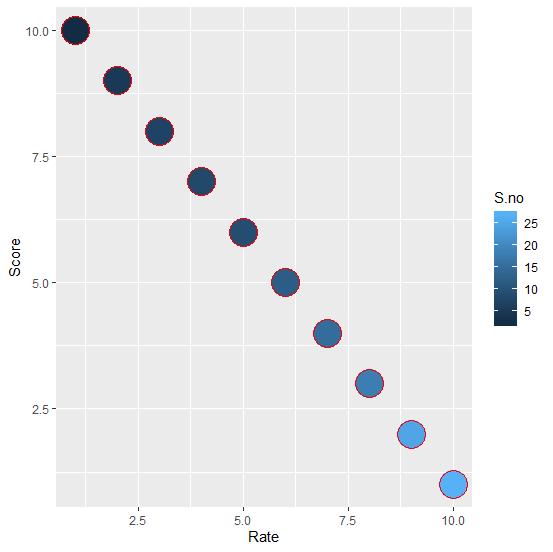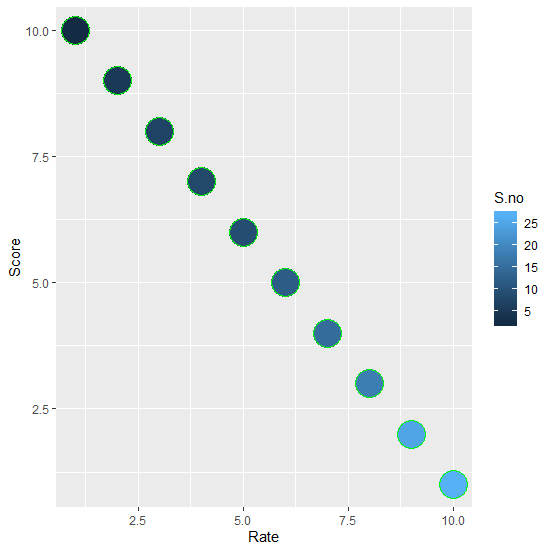# How to change the border color of points in a scatterplot created with ggplot2 in R?

Aesthetics is an essential part of a plot whether it is a scatterplot or any other plot. When we create a scatterplot with ggplot function of ggplot2 package, the border of the points is black if we fill the points with the sequence of a color but we can change these borders to any other color by using colour argument.

## Example

Consider the below data frame

Rate <-1:10
Score <-10:1
S.no <-c(2,5,7,8,9,12,15,18,25,27)
df <-data.frame(Rate,Score,S.no)
df

## Output

Rate Score S.no
1 1 10 2
2 2 9 5
3 3 8 7
4 4 7 8
5 5 6 9
6 6 5 12
7 7 4 15
8 8 3 18
9 9 2 25
10 10 1 27
> library(ggplot2)

Creating the scatterplot without defining border color −

ggplot(df,aes(Rate,Score))+geom_point(aes(fill=S.no),pch=21,size=10)

## OutputCreating the scatterplot with red border color −

ggplot(df,aes(Rate,Score))+geom_point(aes(fill=S.no),pch=21,size=10,colour="red")

## OutputCreating the scatterplot with green border color −

ggplot(df,aes(Rate,Score))+geom_point(aes(fill=S.no),pch=21,size=10,colour="green")


## Output